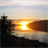# New to Qlik Sense

If you’re new to Qlik Sense, start with this Discussion Board and get up-to-speed quickly.

Announcements
Skip the ticket, Chat with Qlik Support instead for instant assistance.
cancel
Showing results for
Did you mean:Contributor II

## how to use combine rangeavg with distinct

Hi all,

My set analysis is used to calculate average of range unique value. like my example

Date 1: Value = 100

Date 2: Value = 200

Date 3: Value = 300

Date 4: Value = 100

Date 5: Value = 100

So my result what i want to show is:

Date 1: 100

Date 2: (200+100)/2 = 150

Date 3: (100+200+300)/3=200

Date 4: (100+200+300)/3=200 (distinct value 100)

Date 5: (100+200+300)/3=200 (distinct value 100)

I use this analysis: rangeavg(above(sum(distinct value),0,row())) But it not my expectation result